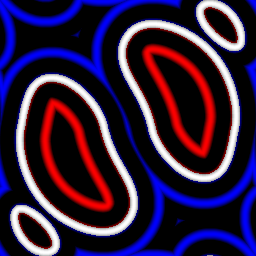The Mask to SDF node generates a signed distance image from an image mask.## Inputs¶

The Mask to SDF node accepts a greyscale image input.

## Outputs¶

The Mask to SDF node generates a signed distance function of the greyscale input mask.

## Parameters¶

The Size used for the image operation to convert the mask into the distance field.

The number of Iterations used in the jump flood operation to calculate the distance field.

Whether the distance field is Tiled.

The Distance function used to calculate the distance field.

## Example images¶